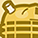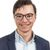# New to Qlik Sense

If you’re new to Qlik Sense, start with this Discussion Board and get up-to-speed quickly.

Announcements
Our next Qlik Insider session will cover new key capabilities. Join us August 11th REGISTER TODAY
cancel
Showing results for
Did you mean:Contributor III

## how to use count expression inside Max function ?

Thank you
It solved half of my problems.

For example one variable vA = 10 , vB = 30 , vC = count(EmpID) these three variables are created using  create new variable option .

Now If I will print it is showing \$(vC) = 45 and is in numeric format(checked by isNum() option )

but  Max(valueList( \$(vA) ,\$(vB) ,\$(vC) )) does not give any solution in chart expression while

Max(valueList( \$(vA) ,\$(vB) )) give  30 as max value in same chart expression. What I got to know using count() inside Max () might be the issue seems though.

Kindly suggest how to achieve same as \$(vC) = count(EmpID) and I need 2nd max of these three variables

Max(valueList( \$(vA) ,\$(vB) ,\$(vC) ),2) but no luck .

as count is present inside \$(vC) , because of same Max () might not work so how to over-ride such scenario as I need comparison .

Labels (1)
• ### Qlik Sense

1 Solution

Accepted SolutionsMVP

Yes, rangemax() is the right function to look for. However, if you need 2nd max from list of values - rangemax() could not help there directly. In such case (ranked value) - your (OP) approach of putting these values in Valuelist() and putting max() outside with rank parameter is a perfect fit. But you are getting an nested aggregation error; for that you could just put '=' sign before your expression in variable definition - which would make the expression evaluation before it is referred in other expression - and you are done!

OR

If you can't change (adding '=' before it) variable definition, you could force evaluate these variables before hand in expression using double dollar expansion like

Max(valueList( \$(=\$(vA)) ,\$(=\$(vB)) ,\$(=\$(vC)) ),2)

5 RepliesPartner

you can't use nested aggregation.
could you provide a sample app to look at?Partner

Try this

max( aggr( count([column_name]), TableDimension))

Thanks and Regards
Mayur IngalePartnerMVP

Yes, rangemax() is the right function to look for. However, if you need 2nd max from list of values - rangemax() could not help there directly. In such case (ranked value) - your (OP) approach of putting these values in Valuelist() and putting max() outside with rank parameter is a perfect fit. But you are getting an nested aggregation error; for that you could just put '=' sign before your expression in variable definition - which would make the expression evaluation before it is referred in other expression - and you are done!

OR

If you can't change (adding '=' before it) variable definition, you could force evaluate these variables before hand in expression using double dollar expansion like

Max(valueList( \$(=\$(vA)) ,\$(=\$(vB)) ,\$(=\$(vC)) ),2)Contributor III
Author

@tresesco  Thank you
finally it worked .Tags
Community Browser